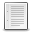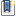File : Details

# Contour3d

Plots 3D contours, iso-surfaces, of function f on 3D lattice x,y,z
Details
Version
10.03
Author
A J Roberts
Maintainer
Tony Roberts
Category
Supported Scilab Version
5.3
Creation Date
April 1, 2010Description
```            contour3d  draws level surfaces of a 3D function
f(x,y,z) on a 3D plot.  The values of f(x,y,z) are to
be in the 3D hypermatrix f at the lattice grid points
defined by 1D or 3D matrices x, y and z.

INPUT  If no arguments are supplied at all, then
contour3d draws a demonstration of nested spheres.
x,y,z: 1D or 3D matrices of coordinates of grid
points in space at which the function f is known.
f: 3D matrix of function values. Set any missing
f-values to %nan.
nf: if nf is simply a number of surfaces to draw,
then a colorbar is also drawn.  However, if nf is
a vector of surface values, then no colorbar. You
can skip one or more colors in the current
colormap by specifying %nan at the corresponding
place in the vector nf of f values---useful for
superimposing iso-surface plots.

Any extra arguments to contour3d are passed on to
plot3d to empower you to set view, legend, and so on.

OUTPUT fvals: vector of set contour values.

Executing contour3d() draws an example.
```Files (1)Miscellaneous file
```Scilab function file as described.
```News (0)Comments (1)Comment from ROUX philippe -- June 30, 2013, 10:35:47 PM
```great tool!  I only find a bug with some nf values see example below

8<--8<--8<--8<--8<--8<--8<--8<--8<--8<--8<--8<--8<--8<--8<--8<--8<--8<--
function t=fq(x,y,z)
u=[x;y;z]
t=(u.')*u // t=x?=y?+z?
endfunction

//compute fq values
x=[-2:0.2:2];y=x;z=x; // the  domain
n=length(x);// number of points
t=zeros(n,n,n)
for i=1:n
for j=1:n
for k=1:n
t(i,j,k)=fq(x(i),y(j),z(k));
end
end
end

clf();
[fvals]=contour3d(x,y,z,t,1);
8<--8<--8<--8<--8<--8<--8<--8<--8<--8<--8<--8<--8<--8<--8<--8<--

nothing is drawn but the surface should be a sphere of center (0,0,0) and radius 1.

Thereis a work around : retry with :

[fvals]=contour3d(x,y,z,t,1+%eps);

then all works perfectly ...```Leave a comment
Login withEmail notifications